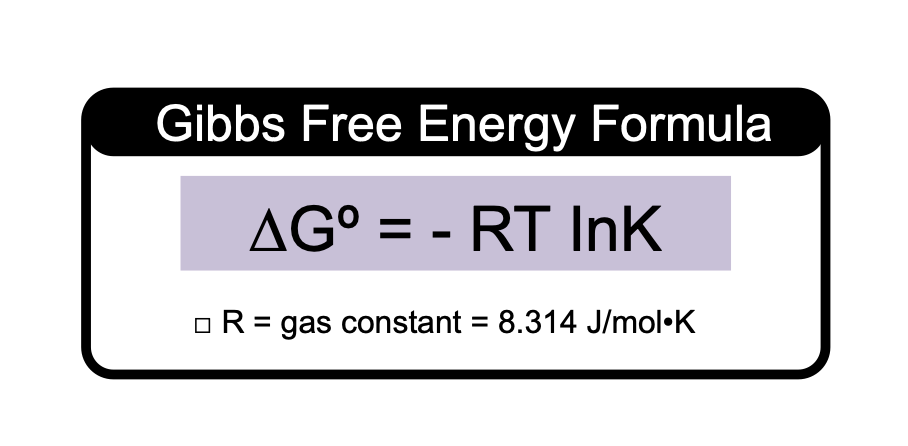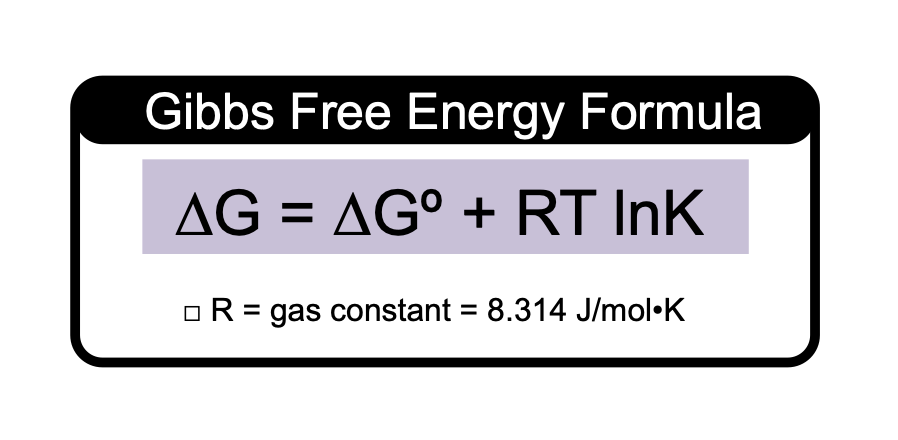Clutch Prep is now a part of Pearson
Ch.17 - Chemical ThermodynamicsWorksheetSee all chapters

# Gibbs Free Energy And Equilibrium

See all sections
Sections
Spontaneous vs Nonspontaneous Reactions
Entropy
Entropy Calculations
Entropy Calculations: Phase Changes
Third Law of Thermodynamics
Gibbs Free Energy
Gibbs Free Energy Calculations
Gibbs Free Energy And Equilibrium

Gibbs Free Energy and the Equilibrium Constant are the two most prominent variables used to determine spontaneity.

###### Gibbs Free Energy-Equilibrium Constant Calculations

Concept #1: If the equilibrium constant and temperature are known for a reaction then the standard Gibbs Free Energy can be calculatedExample #1: A certain reaction takes place at 25ºC and has an equilibrium constant of 2.8 x 104. Determine the Gibbs free energy of the reaction.

Practice: For reaction, Ag2CO3 (s) ⇌ Ag2O (s) + CO2 (g), the ∆Hº = 79.14 kJ/mol, ∆Sº = 167.2 J/K.

Determine the equilibrium constant at which the temperature is 365.1 K.

Concept #2: When standard conditions are not held then the nonstandard version of Gibbs Free Energy must be used.Practice: Consider a hypothetical reaction at 38 ºC, X2 (g) + 2 Y (s) ⇌ 3 Z (g), with a ∆G of −75.8 kJ.

Concentrations of reactants and products: [X2] = 1.4 M, [Y] = 0.34 M, [Z] = 2.6 M. Calculate Keq of the reaction under standard conditions.

Example #2: The given reaction has a ∆Gº of -374 kJ, and partial pressures of SF4, F2, SF6 are 0.63 atm, 0.95 atm, 1.7 atm respectively. Calculate the ∆Grxn for this reaction.

SF4 (g) + F2 (g) → SF6 (g)